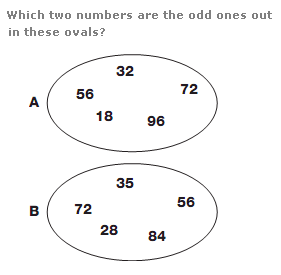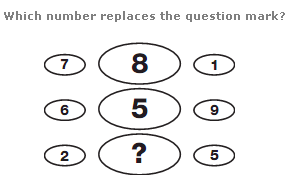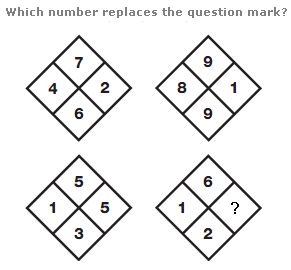# Puzzles - Number puzzles

### Exercise :: Number puzzlesAnswer : A:18 B:72 Explanation : In the first oval, all numbers are divisible by 8, and in the second, they are all divisible by 7.Answer : 5 Explanation : Working through the diagram in rows, find the difference between the left and right hand values, then add 2 to give the value in the central space.Answer : 2 Explanation : In each square, multiply the top and bottom numbers together to give a 2 digit result, and write this result in the left and right hand spaces.